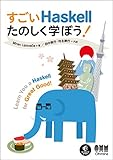# すごいH本 part10

パート10までやってきた自分えらい

## ガード

• 引数の構造で場合分け
• パターンマッチを使う
• 引数の値が満たす性質で場合分け
• ガードを使う

```bmiTell :: Double -> String
bmiTell bmi
| bmi <= 18.5 = "You're underweight, you emo, you!"
| bmi <= 25.0 = "You're supposedly normal. Pfffft, I bet you're ugly!"
| bmi <= 30.0 = "You're fat! Lose some weight, fatty!"
| otherwise   = "You're a whale, congratulations!"
```

```*Main> bmiTell 24.3
"You're supposedly normal. Pfffft, I bet you're ugly!"
*Main> bmiTell 1
"You're underweight, you emo, you!"
*Main> bmiTell 100
"You're a whale, congratulations!"
```
• `| <真偽値式> = <関数本体>` *`if/else`使うよりガード使えとのこと
• `otherwise`でその他のパターンを拾う

その他の例

```max' :: (Ord a) => a -> a -> a
max' a b
| a <= b    = b
| otherwise = a

-- 関数定義時に中置記法を使える
myCompare :: (Ord a) => a -> a -> Ordering
a `myCompare` b
| a == b    = EQ
| a <= b    = LT
| otherwise = GT
```

```*Main> max' 1 3
3
*Main> 1 `myCompare` 3
LT
```

## where

• 計算の途中結果に名前を付けることができる
• ガードの後に書く
```bmiTell' :: Double -> Double -> String
bmiTell' weight height
| bmi <= 18.5 = "You're underweight, you emo, you!"
| bmi <= 25.0 = "You're supposedly normal. Pfffft, I bet you're ugly!"
| bmi <= 30.0 = "You're fat! Lose some weight, fatty!"
| otherwise   = "You're a whale, congratulations!"
where bmi = weight / height ^ 2
```

もうちょっと修正

```bmiTell' :: Double -> Double -> String
bmiTell' weight height
| bmi <= skinny = "You're underweight, you emo, you!"
| bmi <= normal = "You're supposedly normal. Pfffft, I bet you're ugly!"
| bmi <= fat    = "You're fat! Lose some weight, fatty!"
| otherwise     = "You're a whale, congratulations!"
where bmi = weight / height ^ 2
skinny = 18.5
normal = 25.0
fat = 30.0
```

where ブロック内のインデントは揃えないと正しく動かない

## where のスコープ

```greet :: String -> String
greet "Juan" = niceGreeting ++ " Juan!"
greet "Fernando" = niceGreeting ++ " Fernando!"
greet name = badGreeting ++ " " ++ name
where niceGreeting = "Hello! So very nice to see you,"
badGreeting = "Oh! Pffft. It's you."
```

この書き方だと、where で束縛された変数は最後の関数の本体からしか見えない。 `greet name = ...` の部分。そしてコンパイル時に怒られる。

```*Main> :l src/chapter3.hs
[1 of 1] Compiling Main             ( src/chapter3.hs, interpreted )

src/chapter3.hs:56:16: error:
Variable not in scope: niceGreeting :: [Char]

src/chapter3.hs:57:20: error:
Variable not in scope: niceGreeting :: [Char]

```badGreeting :: String
badGreeting = "Oh! Pffft. It's you."

niceGreeting :: String
niceGreeting = "Hello! So very nice to see you,"

greet :: String -> String
greet "Juan" = niceGreeting ++ " Juan!"
greet "Fernando" = niceGreeting ++ " Fernando!"
greet name = badGreeting ++ " " ++ name
```

こんな感じで関数の外で定義してあげないと参照できない

```*Main> greet "Juan"
"Hello! So very nice to see you, Juan!"
*Main> greet "tokoyax"
"Oh! Pffft. It's you. tokoyax"
```

## where の中でパターンマッチ

```initials :: String -> String
initials firstname lastname = [f] ++ ". " ++ [l] ++ "."
where (f:_) = firstname
(l:_) = lastname
```

## where の中で関数定義

```calcBmis :: [(Double), (Double)] -> [Double]
calcBmis xs = [bmi w h | (w, h) <- xs]
where bmi weight height = weight / height ^ 2
```